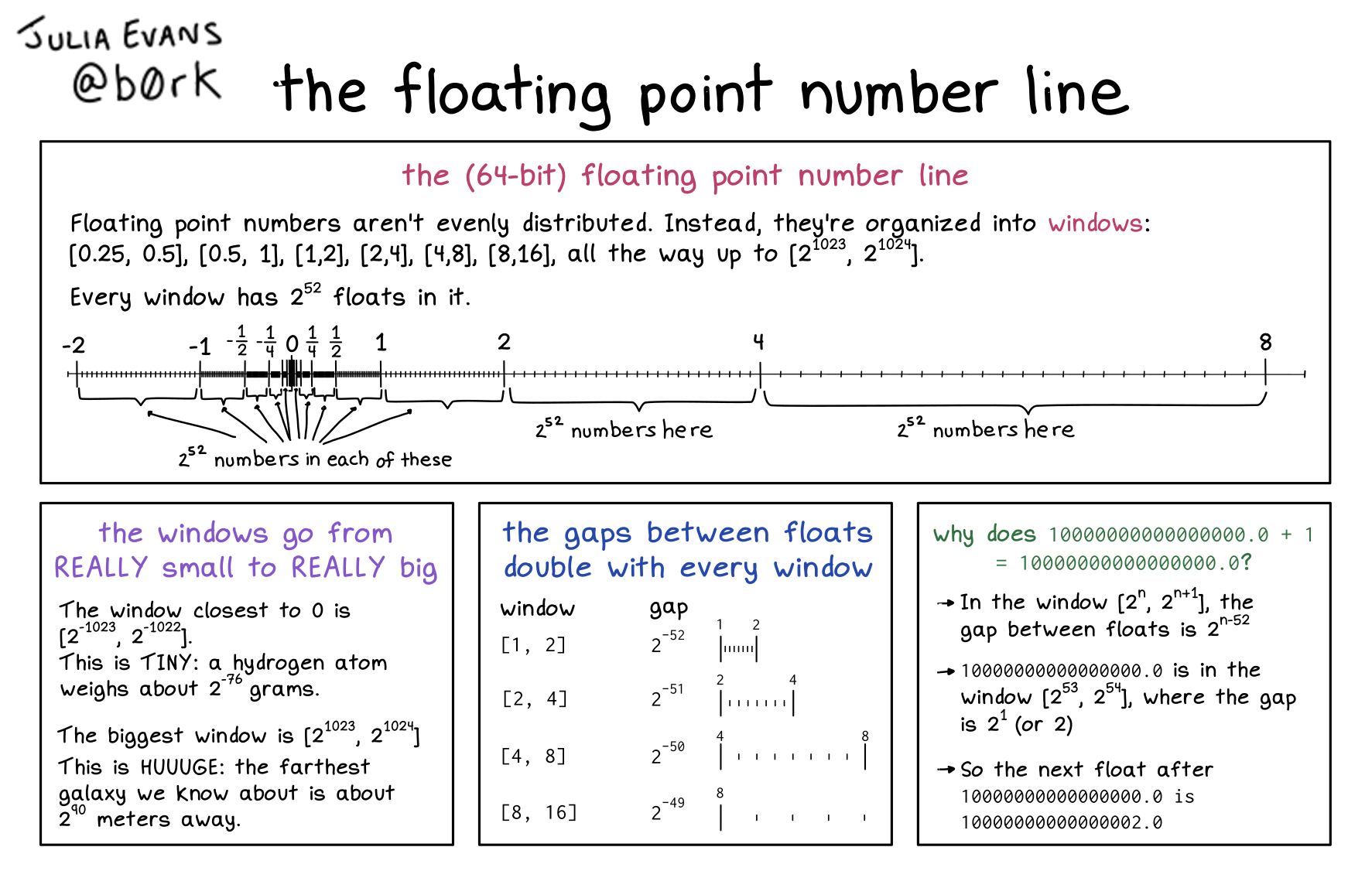Here's a preview from my zine, How Integers and Floats Work! If you want to see more comics like this, sign up for my saturday comics newsletter or browse more comics!subtitle: the 64-bit number line

Floating point numbers aren’t evenly distributed. Instead, between each power of 2 (from 2^-1023 to 2^1023), there are 2^52 numbers, evenly spaced.

There 2^52 numbers between 2 and 4, between 4 and 8, -12 and -14, 1/16/ and 18, etc.

floating point numbers are represented with 3 integers:

1. sign (+ or -, 0 is + and 1 is -)
2. exponent (the power of 2
3. significand (how far it is between that power of 2 and the next one)

here’s how to calculate the exponent and significand for 8.5:

1. find the powers of 2 that sound 8.5 (8 and 16)
2. calculate the exponent: 8 = 2^3, so the exponent is 3
3. calculate the significand:
• 8.5 is 116 of the way between 8 and 16
• rewrite 116 as a fraction of 2^52, so 2^48 / 2^52
• So the significand is 2^48

# Saturday Morning Comics!